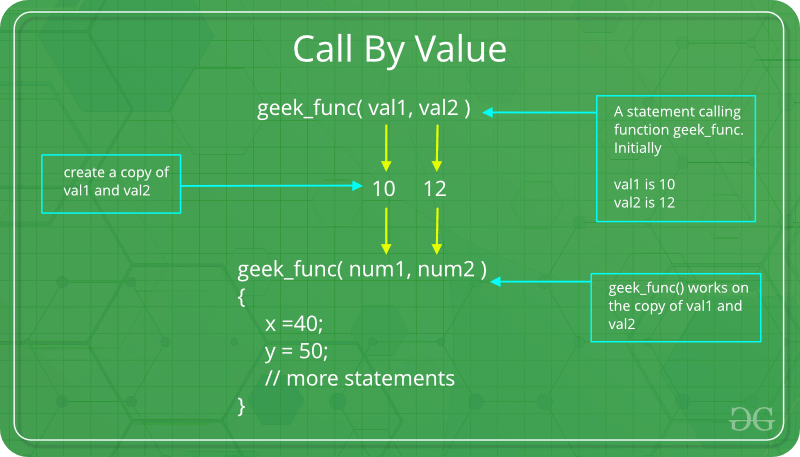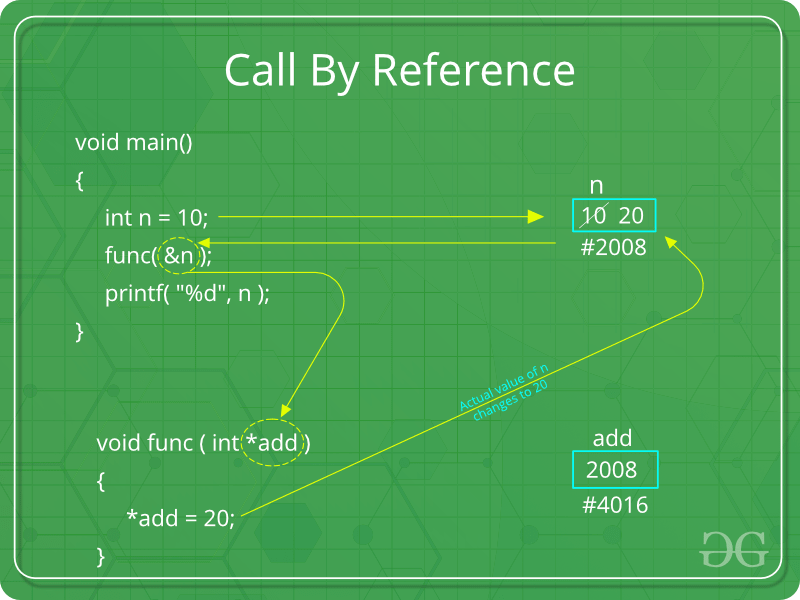GeeksforGeeks App
Open AppBrowser
Continue

# Parameter Passing Techniques in C/C++

There are different ways in which parameter data can be passed into and out of methods and functions. Let us assume that a function B() is called from another function A(). In this case A is called the “caller function” and B is called the “called function or callee function”. Also, the arguments which A sends to B are called actual arguments and the parameters of B are called formal arguments.

Terminology

• Formal Parameter : A variable and its type as they appear in the prototype of the function or method.
• Actual Parameter : The variable or expression corresponding to a formal parameter that appears in the function or method call in the calling environment.
• Modes:
• IN: Passes info from caller to callee.
• OUT: Callee writes values in caller.
• IN/OUT: Caller tells callee value of variable, which may be updated by callee.

Important methods of Parameter Passing

1. Pass By Value: This method uses in-mode semantics. Changes made to formal parameter do not get transmitted back to the caller. Any modifications to the formal parameter variable inside the called function or method affect only the separate storage location and will not be reflected in the actual parameter in the calling environment. This method is also called as call by value.## C

 `// C program to illustrate``// call by value``#include `` ` `void` `func(``int` `a, ``int` `b)``{``    ``a += b;``    ``printf``(``"In func, a = %d b = %d\n"``, a, b);``}``int` `main(``void``)``{``    ``int` `x = 5, y = 7;`` ` `    ``// Passing parameters``    ``func(x, y);``    ``printf``(``"In main, x = %d y = %d\n"``, x, y);``    ``return` `0;``}`

## CPP

 `// C++ program to illustrate``// call by value``#include ``using` `namespace` `std;`` ` `void` `func(``int` `a, ``int` `b)``{``    ``a += b;``    ``cout <<``"In func, a = "` `<< a << ``" b = "``<< b << endl;``}``int` `main(``void``)``{``    ``int` `x = 5, y = 7;`` ` `    ``// Passing parameters``    ``func(x, y);``    ``cout << ``"In main, x = "` `<< x << ``" y = "` `<< y;``    ``return` `0;``}`` ` `// This code is contributed by shivanisinghss2110`

Output:

```In func, a = 12 b = 7
In main, x = 5 y = 7
```

Languages like C, C++, Java support this type of parameter passing. Java in fact is strictly call by value.
Shortcomings:

• Inefficiency in storage allocation
• For objects and arrays, the copy semantics are costly
2. Pass by reference(aliasing): This technique uses in/out-mode semantics. Changes made to formal parameter do get transmitted back to the caller through parameter passing. Any changes to the formal parameter are reflected in the actual parameter in the calling environment as formal parameter receives a reference (or pointer) to the actual data. This method is also called as call by reference. This method is efficient in both time and space.## C

 `// C program to illustrate``// call by reference``#include `` ` `void` `swapnum(``int``* i, ``int``* j)``{``    ``int` `temp = *i;``    ``*i = *j;``    ``*j = temp;``}`` ` `int` `main(``void``)``{``    ``int` `a = 10, b = 20;`` ` `    ``// passing parameters``    ``swapnum(&a, &b);`` ` `    ``printf``(``"a is %d and b is %d\n"``, a, b);``    ``return` `0;``}`

## CPP

 `// C++ program to illustrate``// call by reference``#include ``using` `namespace` `std;`` ` `void` `swapnum(``int``* i, ``int``* j)``{``    ``int` `temp = *i;``    ``*i = *j;``    ``*j = temp;``}`` ` `int` `main(``void``)``{``    ``int` `a = 10, b = 20;`` ` `    ``// passing parameters``    ``swapnum(&a, &b);`` ` `    ``cout <<``"a is "` `<< a <<``" and b is "``<< b;``    ``return` `0;``}`` ` `// this code is contributed by shivanisinghss2110`

Output:

```a is 20 and b is 10
```

C and C++ both support call by value as well as call by reference whereas Java doesn’t support call by reference.
Shortcomings:

• Many potential scenarios can occur
• Programs are difficult to understand sometimes

Other methods of Parameter Passing

These techniques are older and were used in earlier programming languages like Pascal, Algol and Fortran. These techniques are not applicable in high level languages.

1. Pass by Result:This method uses out-mode semantics. Just before control is transferred back to the caller, the value of the formal parameter is transmitted back to the actual parameter. This method is sometimes called call by result. In general, pass by result technique is implemented by copy.
2. Pass by Value-Result: This method uses in/out-mode semantics. It is a combination of Pass-by-Value and Pass-by-result. Just before the control is transferred back to the caller, the value of the formal parameter is transmitted back to the actual parameter. This method is sometimes called as call by value-result
3. Pass by name : This technique is used in programming language such as Algol. In this technique, symbolic “name” of a variable is passed, which allows it both to be accessed and update.
Example:
To double the value of C[j], you can pass its name (not its value) into the following procedure.

```procedure double(x);
real x;
begin
x:=x*2
end;
```

In general, the effect of pass-by-name is to textually substitute the argument in a procedure call for the corresponding parameter in the body of the procedure.
Implications of Pass-by-Name mechanism:

• The argument expression is re-evaluated each time the formal parameter is passed.
• The procedure can change the values of variables used in the argument expression and hence change the expression’s value.

This article is contributed by Krishna Bhatia. If you like GeeksforGeeks and would like to contribute, you can also write an article using contribute.geeksforgeeks.org or mail your article to contribute@geeksforgeeks.org. See your article appearing on the GeeksforGeeks main page and help other Geeks.